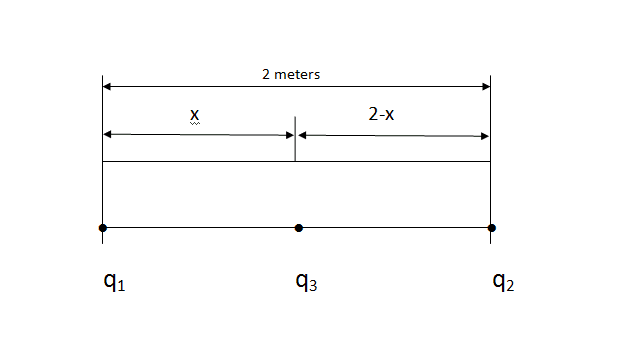# Find the distance x using Coulomb's law.

bllnsr

## Homework Statement

There are 3 charges q1,q2 & q3. Charge q1 is 1.67x10^-10 C and charge q2 is 9.13x10^-6 C. There is no net force on charge q3. x is the distance between q1 and q3 & (2-x) is the distance between q3 and q2. Distance between charge q1 and q2 is 2 meters. Find the distance x.

figure: -Coulomb's law.

## The Attempt at a Solution

I am getting a very weird quadratic equation and i don't even know that if I am solving it right. Any help would be good. I just want to know the steps to solve it.

voko
What is the equation you get and how do you get it?

Mentor
Look at the size of the charges q1 and q3. Which of these do you think the charge q2 will be located closer to at equilibrium? Do you think that q2 will be very, very, very close to q1 or q3?

bllnsr
I solved it like this:-

$Fq_3q_1 - Fq_3q_2 = 0$

$Kq_3q_1/x^2 - Kq_3q_2/(2-x)^2 = 0$

$Kq_3$ is common and will become zero.

By putting values and further solving it I get quadratic equation.

My answer is $x = -3.9914$ & $x = -4.0086$

voko
$$q_1x^2 = q_2(2 - x)^2 \\ \frac {q_1} {q_2} = \frac {(2 - x)^2} {x^2} \\ \frac {q_1} {q_2} = (\frac {2} {x} - 1)^2 \\ \sqrt {\frac {q_1} {q_2}} = \frac {2} {x} - 1$$ Note that the positive sign was selected in front of the radical. This is because the problem requires that $\frac {2} {x} - 1 > 0$.

Steenrod
@voko, how did you write the equation?

I do not know how to use LaTex on physics forums. I tried using $<code>$ but it does not seem to work well.

voko
Click the "quote" button on my message, and you will see the code.

bllnsr
@voko your solution gives 1.99(2) answer and if I put 2 in equation :-

$x+2-x = 2$

x = 2 verifies it so it means the answer is correct right?

Last edited:
voko
Well, $x + 2 - x = 2$ is true for any x, so I don't see how that can verify anything. I am not even sure how you get that expression in the first place. x = .5 would mean that $$\sqrt{\frac {q_1} {q_2}} = \frac 2 {0.5} - 1 = 3$$ which is not true, because $$\sqrt{\frac {q_1} {q_2}} = \sqrt{\frac {1.67\cdot 10^{-10} C} {9.13 \cdot 10^{-6} C} }= \sqrt {1.83 \cdot 10^{-5} } = 4.28 \cdot 10^{-3}$$

bllnsr
Oh yes it is true for any value of x my mistake

Mentor
$$q_1x^2 = q_2(2 - x)^2 \\ \frac {q_1} {q_2} = \frac {(2 - x)^2} {x^2} \\ \frac {q_1} {q_2} = (\frac {2} {x} - 1)^2 \\ \sqrt {\frac {q_1} {q_2}} = \frac {2} {x} - 1$$ Note that the positive sign was selected in front of the radical. This is because the problem requires that $\frac {2} {x} - 1 > 0$.

It looks like you made a bit of an algebra error. The x2 and the (x-2)2 should be in the denominator. As I noted in my earlier reply, q3 is going to be very close to one of the other charges. If q3 is positive, because of the large ratio of q2/q1, q3 will be very close to x = 0, and x can be neglected relative to the 2 in the term (x-2). This greatly simplifies solving for x.

voko
It looks like you made a bit of an algebra error.
Indeed.

Last edited:
voko
Blast. I just realized that I mistyped the original equation. It should have been $$\frac {q_1} {x^2} = \frac {q_2} {(2 - x)^2}$$ which then gives $$\frac {(2 - x)^2} {x^2} = \frac {q_2} {q_1} \\ \frac {2} {x} - 1 = \sqrt {\frac {q_2} {q_1}} \\ x = \frac {2} {\sqrt {\frac {q_2} {q_1}} + 1}$$ which indeed confirms that x should be very small.

bllnsr
@voko x is $8.517*10^{-3}$

voko
That's what I get as well. Sorry for the initial confusion.